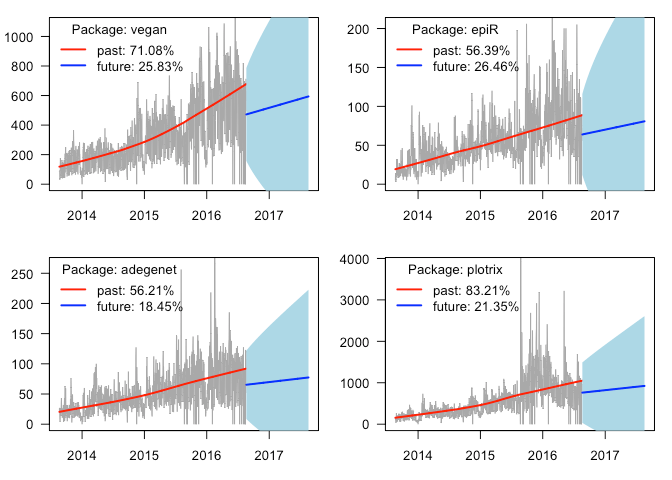[This article was first published on Peter Solymos - R related posts, and kindly contributed to R-bloggers]. (You can report issue about the content on this page here)
Want to share your content on R-bloggers? click here if you have a blog, or here if you don't.

This post was prompted by this blog about using the cranlogs package by Gabor Csardi. But my own interest
as long time package developer dates back to this post by Ben Bolker. I like to see that
my packages
are being used. So I thought why stop at counting downloads and plotting
the past. Why not predict into the future?

The R language has seen steady growth over the past decade in terms of its
use relative to other programming languages
and the number of available extension packages,
both reflecting increasing community engagement.
It is thus interesting to have a look at how individual
packages perform over time based on

As the R user community expands, the disparity between
more and less popular packages is expected to increase.
A handful of packages
are on top of the CRAN download chart harvesting most of the attention
of novice R users.
is not necessarily the best measure of overall impact, more like a
measure that is easiest to quantify.
At least I like to think that 100 downloads with 10 users is way better than 100 downloads with a single user. These two scenarios are hard to tell apart though.

So I wanted to look at temporal patterns in the past 3 years’ download
statistics from RStudio CRAN mirror
for individual R packages. I quantified past trend based
on fitting a log-linear `glm` model to counts vs. dates.
Then I used the forecast package by Rob Hyndman to make short-term forecast
based on an additive non-seasonal exponential smoothing model. Here is the `plot_pkg_trend` function:

```library(cranlogs)
library(forecast)

plot_pkg_trend <-
function(pkg)
{
op <- par(mar = c(3, 3, 1, 1) + 0.1, las = 1)
on.exit(par(op))
## grab the data
x\$date <- as.Date(x\$date)
x\$std_days <- 1:nrow(x) / 365
## past trend
m <- glm(count ~ std_days, x, family = poisson)
tr_past <- round(100 * (exp(coef(m)[2L]) - 1), 2)
## future trend
s <- ts(x\$count)
z <- ets(s, model = "AAN")
f <- forecast(z, h=365)
f\$date <- seq(as.Date("2016-08-20"), as.Date("2017-08-19"), 1)
tr_future <- round(100 * (f\$mean[length(f\$mean)] / f\$mean[1L] - 1), 2)
## plot
plot(count ~ date, x, type = "l", col = "darkgrey",
ylab = "", xlab = "",
ylim = c(0, quantile(x\$count, 0.999)),
xlim = c(x\$date[1L], as.Date("2017-08-19")))
lines(lowess(x\$date, x\$count), col = 2, lwd = 2)
polygon(c(f\$date, rev(f\$date)),
c(f\$upper[,2L], rev(f\$lower[,2L])),
border = NA, col = "lightblue")
lines(f\$date, f\$mean, col = 4, lwd = 2)
legend("topleft", title = paste("Package:", pkg), bty = "n",
col = c(2, 4), lwd = 2, cex = 1,
legend = c(paste0("past: ", tr_past, "%"),
paste0("future: ", tr_future, "%")))
## return the data
invisible(x)
}
```

Next, we list the top 10 R packages from the last month:

```cran_top_downloads("last-month")
##    rank  package  count       from         to
## 1     1     Rcpp 221981 2016-07-23 2016-08-21
## 2     2   digest 181333 2016-07-23 2016-08-21
## 3     3  ggplot2 170577 2016-07-23 2016-08-21
## 4     4     plyr 163590 2016-07-23 2016-08-21
## 5     5  stringi 154918 2016-07-23 2016-08-21
## 6     6  stringr 153917 2016-07-23 2016-08-21
## 7     7 jsonlite 152040 2016-07-23 2016-08-21
## 8     8 magrittr 139596 2016-07-23 2016-08-21
## 9     9     curl 134026 2016-07-23 2016-08-21
## 10   10   scales 130887 2016-07-23 2016-08-21
```

The top package was Rcpp by Dirk Eddelbuettel et al. and
here is the daily trend plot with percent annual trend estimates:

```plot_pkg_trend("Rcpp")
```The increase is clear with increasing day-to-day variation
suggesting that the log-linear model might be appropriate.
Steady increase is predicted (the blue line starts at the
last observed data point, whereas the
red line is a locally weighted `lowess` smoothing curve on
past data).
The light blue 95% prediction
intervals nicely follow the bulk of the zig-zagging in the
observed trend.

Now for my packages, here are the ones I use most often:

```op <- par(mfrow = c(2, 2))
plot_pkg_trend("pbapply")
plot_pkg_trend("ResourceSelection")
plot_pkg_trend("dclone")
plot_pkg_trend("mefa4")
par(op)
```Two of them increasing nicely, the other two are showing
some leveling-off. And finally,
results for some packages that I am contributing to:

```op <- par(mfrow = c(2, 2))
x <- plot_pkg_trend("vegan")
x <- plot_pkg_trend("epiR")
x <- plot_pkg_trend("plotrix")
par(op)
```My approach as outlined here works for packages that have been with us
since 2013. However, there are packages that went extinct (orphaned,
excluded from CRAN, etc.) or appeared after 2013.
These need to be handled with care when comparing across
many packages or changing the time horizon.
If you want to take the idea presented here a step further,